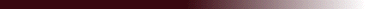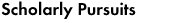## "On the effect of boundary conditions on the multifractal statistics of incompressible turbulence"

### In an earlier paper, the multifractal spectrum has been related to the history and dynamics of the Navier-Stokes flow in an infinite domain. The effect of boundary conditions is now included. The difficulty of distributing the effect of boundary conditions among the wavelet components of the velocity and pressure fields is overcome through the use of Green's functions. The wavelet transforms of Green's functions for the diffusion and Poisson equations are calculated, and are found to be wavelets and combinations of wavelets. In consequence, the integral equation for the velocity field in the (Gaussian) wavelet representation is easily modified, and the formal construction of D_q proceeds as in the case of free turbulence.

IMA Conference on Multiscale Stochastic Processes Analyzed using multifractals and wavelets, Cambridge UK, March 29-31, 1993.

Unpublished. The main results appear in appendix to my `Hamiltonian' paper

Jacques Lewalle, jlewalle@syr.edu